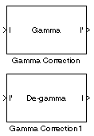# Gamma Correction

Apply or remove gamma correction to or from image or video stream

• Library:
• Computer Vision Toolbox / Conversions

•## Description

The Gamma Correction block applies or removes gamma correction to or from an image or video stream.

## Ports

### Input

expand all

Input image, specified as an M-by-N matrix or an M-by-N-by-C array, where C is the number of color channels in the input image.

#### Dependencies

To enable this port, set the Operation parameter to `Gamma`.

Data Types: `single` | `double` | `int8` | `int16` | `uint8` | `uint16` | `Boolean` | `fixed point`

Gamma-corrected input image, specified as an M-by-N matrix or an M-by-N-by-C array, where C is the number of color channels in the input image.

#### Dependencies

To enable this port, set the Operation parameter to `De-gamma`.

Data Types: `single` | `double` | `int8` | `int16` | `uint8` | `uint16` | `Boolean` | `fixed point`

### Output

expand all

Gamma-corrected output image, returned as an M-by-N matrix or an M-by-N-by-C array, where C is the number of color channels.

#### Dependencies

To enable this port, set the Operation parameter to `Gamma`.

Data Types: `single` | `double` | `int8` | `int16` | `uint8` | `uint16` | `Boolean` | `fixed point`

Gamma-removed output image, returned as an M-by-N matrix or an M-by-N-by-C array, where C is the number of color channels.

#### Dependencies

To enable this port, set the Operation parameter to `De-gamma`.

Data Types: `single` | `double` | `int8` | `int16` | `uint8` | `uint16` | `Boolean` | `fixed point`

## Parameters

expand all

Specify the gamma operation the block performs.

• `Gamma` — Apply gamma correction to the input image.

• `De-gamma` — Remove gamma correction from the input image.

Specify the gamma as a value greater than or equal to 1. A value less than 1 encodes luminance values in image and a value greater than 1 decodes luminance values in image, represented as gamma compression and gamma expansion respectively.

Select this parameter to define the gamma curve as a linear segment near the origin. For more information, see Gamma Correction Method.

Specify a scalar break point value, which indicates the I-axis value at the end of the gamma curve linear segment. For more information, see Gamma Correction Method.

#### Dependencies

To enable this parameter, select the Linear segment parameter.

## Block Characteristics

 Data Types `double` | `fixed pointa` | `integera` | `single` Multidimensional Signals `yes` Variable-Size Signals `yes` a Supports word lengths less than or equal to 16 bits.

## Algorithms

expand all

 Poynton, Charles A. Digital Video and HDTV: Algorithms and Interfaces. Morgan Kaufmann Series in Computer Graphics and Geometric Modeling. Amsterdam ; Boston: Morgan Kaufmann Publishers, 2003.